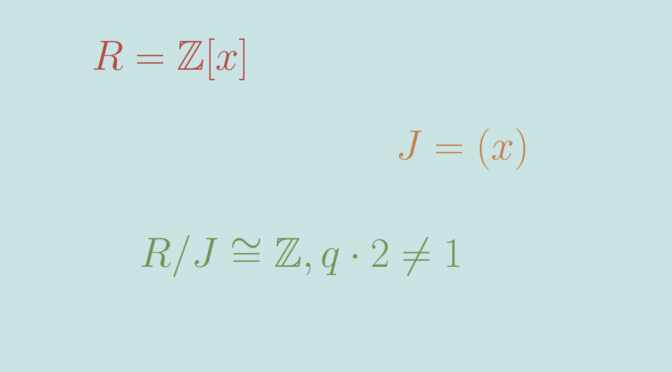# A prime ideal that is not a maximal ideal

Every maximal ideal is a prime ideal. The converse is true in a principal ideal domain – PID, i.e. every nonzero prime ideal is maximal in a PID, but this is not true in general. Let’s produce a counterexample.

$$R= \mathbb Z[x]$$ is a ring. $$R$$ is not a PID as can be shown considering the ideal $$I$$ generated by the set $$\{2,x\}$$. $$I$$ cannot be generated by a single element $$p$$. If it was, $$p$$ would divide $$2$$, i.e. $$p=1$$ or $$p=2$$. We can’t have $$p=1$$ as it means $$R = I$$ but $$3 \notin I$$. We can’t have either $$p=2$$ as it implies the contradiction $$x \notin I$$. The ideal $$J = (x)$$ is a prime ideal as $$R/J \cong \mathbb Z$$ is an integral domain. Since $$\mathbb Z$$ is not a field, $$J$$ is not a maximal ideal.